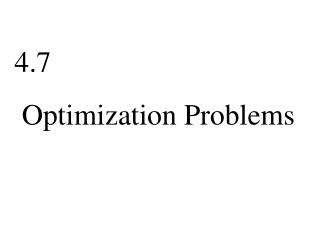Download Presentation4.7 Optimization Problems

# 4.7 Optimization Problems - PowerPoint PPT PresentationDownload Presentation## 4.7 Optimization Problems

- - - - - - - - - - - - - - - - - - - - - - - - - - - E N D - - - - - - - - - - - - - - - - - - - - - - - - - - -
##### Presentation Transcript

1. 4.7 Optimization Problems

2. Steps to follow for max and min problems. (a) Draw a diagram, if possible. (b) Assign symbols to unknown quantities. (c) Assign a symbol,Q, to the quantity to be maximized or minimized. (d) Express Q in terms of the other symbols. (e) Eliminate all but one unknown symbol, say x, from Q.

3. (f) Find the absolute maximum or minimum of Q= f (x). (iii) Use the first derivative (iv) Does the absolute max or min

4. 1. Example: Solution: =˃p(x)=x(x-100)

5. (i) Domain of P(x): all real numbers. (ii) Critical numbers of P(x) P′(x)= (iii) First Derivative Test P′(x)

6. P(50) is an absolute min value.

7. Graph:

8. 2. Example: A farmer wants to fence a rectangular enclosure for his horses and then divide it into thirds with fences parallel to one side of the rectangle. If he has 2000m of fencing, find the area of the largest rectangle that can be enclosed. Solution:

9. (i) Domain of A(l):

10. (ii) Critical numbers of A(l) By the first derivative test we can show that A(500) is the max value of A,

11. When l=500, max area of the rectangle =

12. Graph:

13. 3. Example: x=y2 that is closest to the poit (0,3). Let D denote the distance from (0,3) to any point (x,y) on the parabola.

14. D(y)= Find the value of y which makes D(y) (i) Domain of D(y): all real numbers > 0.

15. (iii) First Derivative Test D′(y) By the first derivative test D(y)

16. Graph:

17. (i) Domain of V(y):

18. (ii) Critical numbers of V(y) 2π r2 -3y2 By the first derivative test

19. 5. Example: Mountain Beer sells its beer in an aluminum can in the shape of a right- circular cylinder. The volume of each can What should the dimension of the can be in order to minimize the amount of aluminum used? Solution: and

20. r h Surface Area,

21. S(r)= (i) Domain of S(r) (ii) Critical numbers of S(r) S′(r)=

22. S′(r)=0 =˃ (iii) First Derivative Test S′(r)= S′(r) S(r) S(r) has its absolute minimum value

23. Graph: